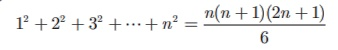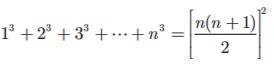Home | | Maths 10th Std | Points to Remember

# Points to Remember

Numbers and Sequences: Euclid’s division lemma, Fundamental theorem of arithmetic, Arithmetic Progression, Geometric Progression, Special Series

Points to Remember

### Euclid’s division lemma

If a and b are two positive integers then there exist unique integers q and r such that a = bq + r, 0 r < |b|

### Fundamental theorem of arithmetic

Every composite number can be expressed as a product of primes and this factorization is unique except for the order in which the prime factors occur.

Arithmetic Progression

(i) Arithmetic Progression is aa + da + 2da + 3d,.nth term is given by tn  = a + (n 1)d

(ii) Sum to first n terms of an A.P. is(iii) If the last term l (nth  term is given, then Sn)

### Geometric Progression

(i) Geometric Progression is aarar2 ,.,arn1 . nth  term is given by tn= ar n1

(ii) Sum to first n terms of an G.P. is(iii) Suppose r =1 then S n  = na

(iv) Sum to infinite terms of a G.P. a + ar + ar2 +  isWhere –1< r < 1

### Special Series

(i) The sum of first n natural numbers 1 + 2 + 3 +  + n(ii) The sum of squares of first n natural numbers(iii) The sum of cubes of first n natural numbers(iv) The sum of first n odd natural numbers 1 + 3 + 5 + .... ..  + (2n1) = n2

Tags : Numbers and Sequences | Mathematics , 10th Mathematics : UNIT 2 : Numbers and Sequences
Study Material, Lecturing Notes, Assignment, Reference, Wiki description explanation, brief detail
10th Mathematics : UNIT 2 : Numbers and Sequences : Points to Remember | Numbers and Sequences | Mathematics